Courses

# ​Frequency Modulation Electronics and Communication Engineering (ECE) Notes | EduRev

## Communication Theory

Created by: Machine Experts

## Electronics and Communication Engineering (ECE) : ​Frequency Modulation Electronics and Communication Engineering (ECE) Notes | EduRev

The document ​Frequency Modulation Electronics and Communication Engineering (ECE) Notes | EduRev is a part of the Electronics and Communication Engineering (ECE) Course Communication Theory.
All you need of Electronics and Communication Engineering (ECE) at this link: Electronics and Communication Engineering (ECE)

FREQUENCY MODULATION:

Frequency modulation (FM) conveys information over a carrier wave by varying its instantaneous frequency. This is in contrast with amplitude modulation, in which the amplitude of the carrier is varied while its frequency remains constant. In analog applications, the difference between the instantaneous and the base frequency of the carrier is directly proportional to the instantaneous value of the input signal amplitude. Digital data can be sent by shifting the carrier's frequency among a set of discrete values, a technique known as frequency-shift keying.

Frequency modulation can be regarded as phase modulation where the carrier phase modulation is the time integral of the FM modulating signal.

FM is widely used for broadcasting of music and speech, and in two-way radio systems, in magnetic tape recording systems, and certain video transmission systems. In radio systems, frequency modulation with sufficient bandwidth provides an advantage in cancelling naturally-occurring noise. Frequency-shift keying (digital FM) is widely used in data and fax modems.

THEORY:

Suppose the baseband data signal (the message) to be transmitted is xm(t) and the sinusoidal carrier is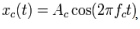, where fc is the carrier's base frequency and Ac is the carrier's amplitude. The modulator combines the carrier with the baseband data signal to get the transmitted signal: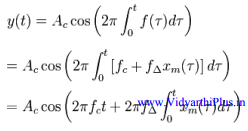In this equation f (T), is the instantaneous frequency of the oscillator and  fΔ is the frequency deviation, which represents the maximum shift away from fc in one direction, assuming xm(t) is limited to the range ±1. Although it may seem that this limits the frequencies in use to f± fΔ, this neglects the distinction between instantaneous frequency and spectral frequency. The frequency spectrum of an actual FM signal has components extending out to infinite frequency, although they become negligibly small beyond a point.

Although it may seem that this limits the frequencies in use to fc ± fΔ, this neglects the distinction between instantaneous frequency and spectral frequency. The frequency spectrum of an actual FM signal has components extending out to infinite frequency, although they become negligibly small beyond a point.

SINUSOIDAL BASEBAND SIGNAL:

While it is an over-simplification, a baseband modulated signal may be approximated by a sinusoidal Continuous Wave signal with a frequency fm. The integral of such a signal is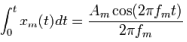Thus, in this specific case, equation (1) above simplifies to: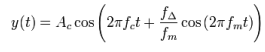where the amplitude  Aof the modulating sinusoid, is represented by the peak deviation (see frequency deviation)fΔ . The harmonic distribution of a sine wave carrier modulated by such a sinusoidal signal can be represented with Bessel functions - this provides a basis for a mathematical understanding of frequency modulation in the frequency domain.

MODULATION INDEX:

As with other modulation indices, this quantity indicates by how much the modulated variable varies around its unmodulated level. It relates to the variations in the frequency of the carrier signal: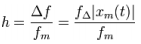where is the highest frequency component present in the modulating signal xm(t), and Δf is the Peak frequency-deviation, i.e. the maximum deviation of the instantaneous frequency from the carrier frequency. If  h << 1, the modulation is called narrowband FM, and its bandwidth is approximately 2fm . If h >>1 , the modulation is called wideband FM and its bandwidth is approximately 2f Δ. While wideband FM uses more bandwidth, it can improve signal-to-noise ratio significantly.

With a tone-modulated FM wave, if the modulation frequency is held constant and the modulation index is increased, the (non-negligible) bandwidth of the FM signal increases, but the spacing between spectra stays the same; some spectral components decrease in strength as others increase. If the frequency deviation is held constant and the modulation frequency increased, the spacing between spectra increases.

Frequency modulation can be classified as narrow band if the change in the carrier frequency is about the same as the signal frequency, or as wide-band if the change in the carrier frequency is much higher (modulation index >1) than the signal frequency.  For example, narrowband FM is used for two way radio systems such as Family Radio Service where the carrier is allowed to deviate only 2.5 kHz above and below the center frequency, carrying speech signals of no more than 3.5 kHz bandwidth. Wide-band FM is used for FM broadcasting where music and speech is transmitted with up to 75 kHz deviation from the center frequency, carrying audio with up to 20 kHz bandwidth.

CARSON'S RULE:

A rule of thumb, Carson's rule states that nearly all (~98%) of the power of a frequency-modulated signal lies within a bandwidth of BT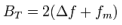where Δf , as defined above, is the peak deviation of the instantaneous frequency f(t) from the center carrier frequency fc.

NOISE QUIETING:

The noise power decreases as the signal power increases, therefore the SNR goes up significantly

MODULATION:

• FM signals can be generated using either direct or indirect frequency modulation
• Direct FM modulation can be achieved by directly feeding the message into the input of a VCO.
• For indirect FM modulation, the message signal is integrated to generate a phase modulated signal. This is used to modulate a crystal controlled oscillator, and the result is passed through a frequency multiplier to give an FM signal.
Offer running on EduRev: Apply code STAYHOME200 to get INR 200 off on our premium plan EduRev Infinity!

,

,

,

,

,

,

,

,

,

,

,

,

,

,

,

,

,

,

,

,

,

;